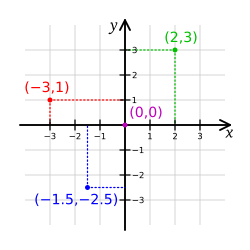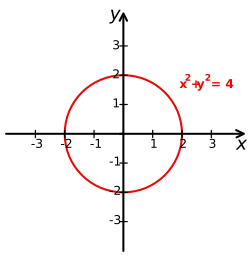# Cartesian coordinate system facts for kids

Kids Encyclopedia Facts
(Redirected from Coordinate plane)Two dimensional Cartesian coordinate system. Four points are marked: (2,3) in green, (-3,1) in red, (-1.5,-2.5) in blue and (0,0), the origin, in violet.

In mathematics, the Cartesian coordinate system is a coordinate system used to place points on a plane using two numbers, usually called the x-coordinate and the y-coordinate. To place the coordinates, two perpendicular lines, called axes (Singular: axis), are drawn. Cartesian coordinates can be used in 3D, by adding a third number, the z-coordinate. Each added coordinate represents a dimension.

Using the Cartesian coordinate system, simple shapes, like parabolas, can be made using algebraic equations. The results of the equations can be plotted (drawn) on the Cartesian grid, and a shape appears. For example, a circle can be made with the equation x² + y² = 4 (see Figure 2).

The name cartesian comes from the French mathematician and philosopher René Descartes (Latin: Cartesius), who worked to merge algebra and Euclidean geometry. This work was important in creating calculus and cartography.

The idea of this system was developed in 1637 in two works by Descartes. In part two of his Discourse on Method, Descartes explains plotting the position of a point or object on a surface, using two perpendicular axes as measuring guides. In La Géométrie, he further explores these ideas.

## Related pagesCartesian coordinate system with the circle of radius 2 centered at the origin marked in red. The equation of the circle is x² + y² = 4.

## Images for kidsCartesian coordinate system Facts for Kids. Kiddle Encyclopedia.